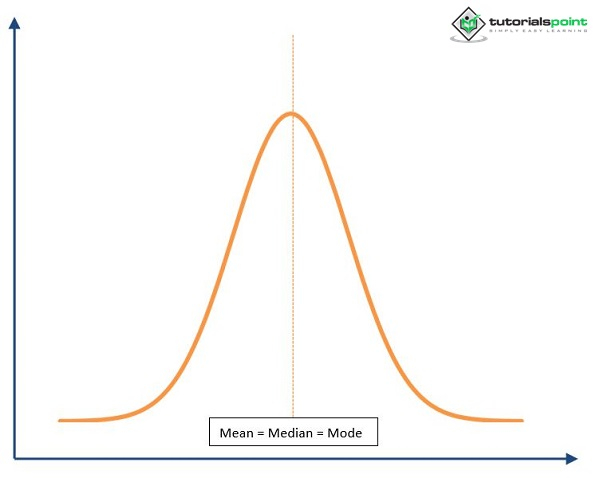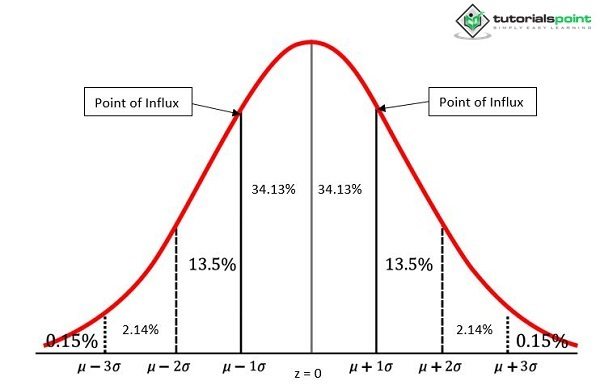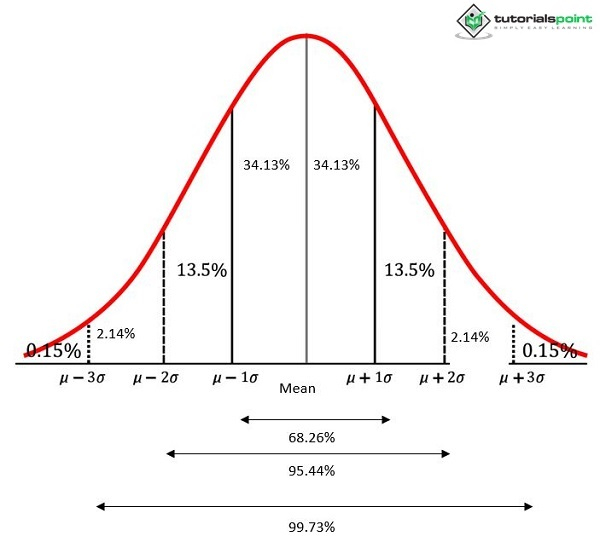# Application of Normal Probability Distribution Technique in Psychology

The literal meaning of normal is average. In psychological studies, sociological studies, and didactics, those who can reach a particular fixed level in qualification and characteristics are termed normal. At the same time, there who are above or below this point are abnormal. Nature has been kind enough to distribute quite equally most attributes like weight, height, intelligence, and the like. As a result, most of us possess average weight, height, intelligence, and such attributes. Quite a few people deviate noticeably from average, either above or below. This holds equally for information gathered through exams, surveys, and experiments in psychology, sociology, and education on a randomly chosen sample or population regarding achievement scores, IQ scores, rating scores, etc. If such a distribution is to be plotted on graph paper, an interesting typical curve would be formed resembling a vertical cross-section of a bell. This bell-shaped curve is referred to as a normal curve.

Laplace and Gauss (1777-1855) derived this curve independently. They worked on experimental errors in physics and astronomy. They found that the resultant error distribution was normal — and thus, the curve of error came to know as the normal error curve or just the Error Curve.

## What is Normal Probability Distribution?

It gives probable score distribution for a continuous variable per the tenets of probability. A mathematician named Abraham de Moivre developed this curve, which has a unilateral bell shape with bilateral symmetry and is built on the tenets of probability. The very tenet of probability is built on the likeliness that an event will occur, and the normal curve depicts this tenet.

## Computation

The plotting of the frequencies (f) of scores of a continuous variable, observed in a sample of a considerably large size, against the respective scores (X) gives a normal distribution curve

A distribution of similar shape is obtained if the relative frequencies (fln), obtained by dividing each observed frequency by the total frequency n, are plotted against the respective standard scores (z-scores) computed from the raw X scores because a linear transformation of X scores derives z-scores; this distribution, however, is known as the normal distribution since its Y ordinate offers the Z-scores relative frequencies or probability, as well as that of the accompanying X scores rather than the observed frequencies.

The general equation mathematically describing the normal probability distribution is written as follows

$\mathrm{y = \frac{n}{\sigma\sqrt{2\Pi}}e^\frac{−x^{2}}{2\sigma^{2}}}$

Where,

$\mathrm{y}$ = frequency

$\mathrm{n}$ = number of observation

$\mathrm{\sigma}$ = standard deviation of distribution

$\mathrm{\Pi}$ = 3.1416 (approximately)

$\mathrm{e}$ = 2.718 (approximately), the base of the Napierian logarithms

$\mathrm{x}$ = deviation of a measurement from the mean

## Example

The sample size is 1000 cases. The test scores' mean value is 14.5, and the standard deviation is 2.5. Assuming normality of distribution, count the number of individuals scoring in the 12−16.

Solution

Both the raw scores 12 and 16 require conversion into z-scores.

z-score equivalent to raw score 12 = $\mathrm{\frac{X − M}{\sigma}}$

= (12−14.5)/2.5

= −2.5/2.5 = −1$\sigma$

z-score equivalent to raw score 16 = (16 − 14.5)/2.5 = 1.5/2.5

= 0.6 $\sigma$

As per the normal curve table, 2257 (out of 10000), i.e., 22.57$\%$ of cases lie between mean and 0.6 $\sigma$. Similarly, between −1 $\sigma$ and mean, 3413, i.e., 34.13$\%$ of cases lie. In this way, it may be easily concluded that 22.57 + 34.13 = 56.7$\%$ or 567 individuals out of 1000 score in the range of 12 − 16.

## Properties

• The normal probability curve is bilaterally symmetrical. The normal curve is bilaterally symmetrical around the vertical axis called ordinate. 50$\%$ of the curves are to the left of the maximum central ordinate, and the other 50$\%$ is to the right. The symmetry about the ordinate at the curve's central point indicates that the curve's slope, shape, and size on the curve's side are alike the other. The following figure shows the right and left halves of the central middle point

Mean = Median = Mode• The normal probability curve is unimodal. The curve has only one apex point, making it unimodal, and it can only have a single mode.

• The maximum ordinate is found near the center. The ordinate's height always peaks at the curve's center or mid-point, equaling 0.3989 on the unit normal curve.

• The normal probability curve is asymptomatic to the X-axis. Asymptotically approaching the X-axis, the curve's height gradually decreases on both ends farther from the middle point but never touches the X-axis. Therefore, its ends extend in the minus infinity ($− \infty$) and plus infinity ($+ \infty$).

• The curve's height decreases equally. There is a symmetrical decrease in the curve's height in either direction from the apex point.

• The points of Influx occur at ±1 standard deviation ($\pm \: 1 \: \sigma$). The change in the normal curve's direction from convex to concave occurs at a place called a point of influx. Perpendicular lines drawn from the two influx points to the X-axis touch the central point at a distance on standard deviation unit from above and below the mean.

• The percentage of the normal curve's total area within two sites of influx is fixed. About 68.26$\%$ of its total area lies in the range of ±1 standard deviation ($\pm$ 1 $\sigma$) unit from the mean• The total area covered by the curve may also be approaching 100$\%$ probability. The total area falling under the curve may be considered to approach 100$\%$ probability, interpreted in terms of standard deviations.• The percentage of the care between successive standard deviations in the normal distribution. Normal distribution serves as a benchmark for kurtosis. It has a mesokurtic character − its percentile coefficient of kurtosis is 0.263.

## Divergence in Normality

When the frequency distribution of test scores is graphically depicted in a histogram or polygon, one readily notices the symmetry or lack of it in the shape of the curve. The three parameters-mean, median, and mode-coincide in the normal curve model; the curve's right and left halves are in perfect balance. Generally, two types of divergence occur in the normal curve - kurtosis and skewness.

## Conclusion

The normal probability curve is held in high significance. It is widely applied for psychological, sociological, and educational measurements. It can be used as a model for computing percentile and percentile rankings, understanding and applying the concept of standard measurement error, and transforming and combining qualitative data. Normal probability distributions have applications that can solve problems such as converting raw scores into z-scores and showing the fractional parts of the normal curve's total area concerning the sigma distances.